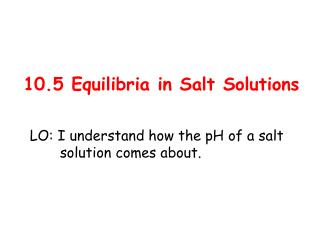DownloadDownload Presentation10.5 Equilibria in Salt Solutions

# 10.5 Equilibria in Salt Solutions

Télécharger la présentation## 10.5 Equilibria in Salt Solutions

- - - - - - - - - - - - - - - - - - - - - - - - - - - E N D - - - - - - - - - - - - - - - - - - - - - - - - - - -
##### Presentation Transcript

1. 10.5 Equilibria in Salt Solutions LO: I understand how the pH of a salt solution comes about.

2. weak Sodium ethanoate is the salt of a acid and a base. strong Sodium ethanoate completely splits into when dissolved in water: ions The pH of sodium ethanoate is . CH3COO-Na+(s) + water CH3COO-(aq) + Na+(aq) + H2O(l) H+(aq) + OH-(aq) CH3COOH(aq) >7 LO: I understand how the pH of a salt solution comes about.

3. strong Ammonium chloride is the salt of a acid and a base. weak Ammonium chloride also completely splits into . when dissolved in water: ions The pH of ammonium chloride is . NH4+Cl-(s) + water NH4+(aq) + Cl-(aq) + H2O(l) OH-(aq) + H+(aq) NH3(aq) + H2O(l) <7 LO: I understand how the pH of a salt solution comes about.

4. strong Sodium chloride is the salt of a acid and a base. strong The pH of sodium chloride is . Na+Cl-(s) + water Na+(aq) + Cl-(aq) Neither ion is involved in an equilibrium. 7 LO: I understand how the pH of a salt solution comes about.